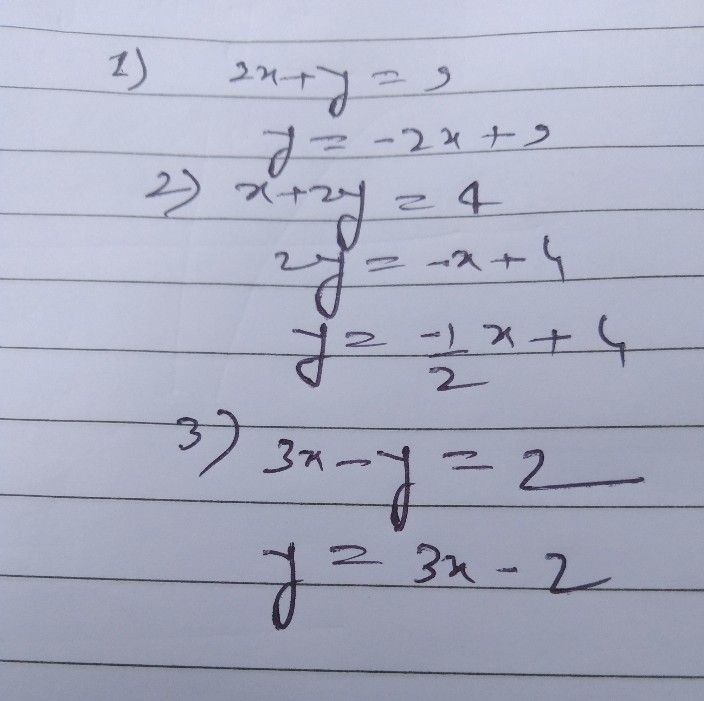Symbol
Problem$A.$ Rewrite the following linear equation in the $om$ $y=mx+b$ $1.$ $2x+y=9$ $2.x+2y=4$ Stnstbsup bnidt $3.3x-y=2$ Rewrite the following linear equation in the $omAx+By=C$ $4$ $y=-2x+6$ nw $5.y=2x+6$ bas 2sldsinev O owi ni noiteU nl
7th-9th grade
Other
Search count: 105
SolutionQanda teacher - jaisi